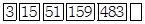Courses

# Test: Numbers - 2

## 10 Questions MCQ Test Mathematics (Maths) for Class 4 | Test: Numbers - 2

Description
This mock test of Test: Numbers - 2 for Class 4 helps you for every Class 4 entrance exam. This contains 10 Multiple Choice Questions for Class 4 Test: Numbers - 2 (mcq) to study with solutions a complete question bank. The solved questions answers in this Test: Numbers - 2 quiz give you a good mix of easy questions and tough questions. Class 4 students definitely take this Test: Numbers - 2 exercise for a better result in the exam. You can find other Test: Numbers - 2 extra questions, long questions & short questions for Class 4 on EduRev as well by searching above.
QUESTION: 1

Solution:
QUESTION: 2

### By what number should the face value of 5 be multiplied in order, to get the place value of 5 in the numeral 24897566?

Solution:

Given number = 24897566

In the given number 5 is in third place.

We have to put two zeros next to five to get the place value of 5.

So, the place value of 5 is 500.

The number should multiplied to 5  = 500/5 = 100.

Hence, 100 should be multiplied with 5 in order to get the place value of 5.

QUESTION: 3

### What least number should be added to 5483855 such that place value of 9 in the resulting numeral becomes 900000?

Solution:

for the number 5483855, place value of hundred thousandth is 400000.

If we can turn this 4 into a 9, place value of hundred thousandth is 900000

So the least number that the place value of hundred thousandth is 900000 will be 5900000. In order to get there you have to add 416145. Which can be obtain by,

5900000 - 5483855

QUESTION: 4

Write smallest six digit number and find its predecessor.

Solution:
QUESTION: 5

What will be the successor of X, if X is the sum of 25689 and 45789?

Solution:
QUESTION: 6

Find the successor of the number which is 2345650 less than the sum of 44777464 and 35976304.

Solution:
QUESTION: 7

Complete the following number series:Solution:
QUESTION: 8

Find the digit which is at the hundred billions place in the 241021032565652.

Solution:
QUESTION: 9

Arrange the following numbers in ascending order: 2467, 2498, 2476, 2746

Solution:
QUESTION: 10

In a numeral, the digit 7 is at ten arabs place. Place value of 7 in the numeral will be:

Solution: